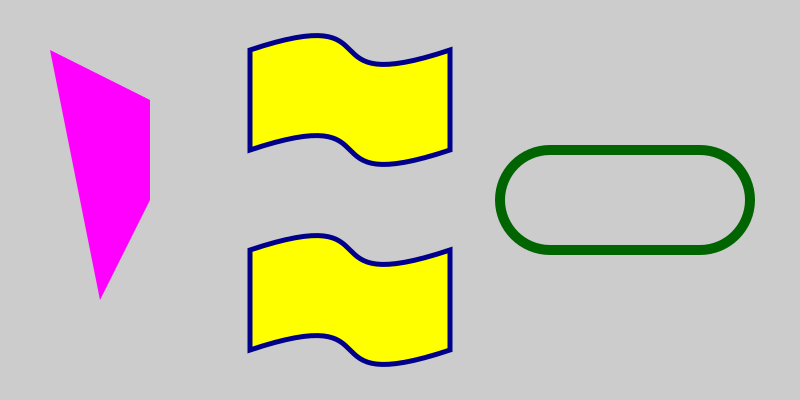# Composite paths in generativepy

Martin McBride, 2020-10-24
Tags generativepy tutorial composite path
Categories generativepy generativepy tutorial

This tutorial shows how to composite paths in generativepy. You should read through the fill and stroke tutorial first, if you haven't already.

generativepy allows you to draw various preset shapes, such as rectangles, circles, and triangles, but of course you can only use these to draw a limited set of shapes.

It also allows you to draw some different line types - straight lines, bezier curves, polylines, and circular/elliptical arcs.

Composite paths provide a way to create new shapes by combining several line types to create new shapes.

## Examples of composite paths

Here are some examples of composite paths:## Composite paths with lines

The magenta polygon on the left of the image shows the basic technique of creating composite paths. Since this is just a normal polygon, we don't really need to do it this way, we could just use a Polygon object. In fact we draw this same shape in the polygons tutorial.

But in this case we will draw the polygon by composing lines, purely to illustrate composite paths. Here is the code:

        Line(ctx).of_start_end((50, 50), (150, 100)).add()
Line(ctx).of_end((100, 300))\
.extend_path(close=True)\
.fill(Color('magenta'))


Before we look at this in detail, we need to quickly look at how the context, ctx works with paths. The context stores a path internally, and we can add to that path with more code. The context also maintains a current point. The current point starts at (0, 0), but whenever we draw anything, the current point is set to the end of the last thing we drew. This allows us to join lines and curves to make shapes.

The code creates a single shape from three Line objects like this:

• The first Line creates a line from (50, 50) to (150, 100), in the normal way. But instead of stroking the line, we call add, which simply adds the line to the path that is stored by the context.
• The second Line is created using of_end - this only defines the end point, not that start point. It then calls extend_path. This means that instead of creating a new path for this line, we will add it to the existing path. This line will start at the current point ie (150, 100) where the previous line ended, and end at (150, 200). Once again we call add to add the line to the path without drawing it.
• The third Line is also created using of_end. It then calls extend_path, but this time with close set to True, to close the shape. This line will start at the current point ie (150, 200) where the previous line ended, and end at (100, 300). A final line will be added to close the polygon. This time we call fill to draw the shape.

## Composite paths with beziers

The top waving flag shape, in the centre of the image, is composed of a mix of bezier curves and lines:

        Bezier(ctx).of_abcd((250, 50), (400, 0), (300, 100), (450, 50))\
Bezier(ctx).of_bcd((300, 200), (400, 100), (250, 150))\
.extend_path(close=True)\
.fill(Color('yellow'))\
.stroke(Color('darkblue'), 5)


In this case:

• The first element Bezier creates a curve using of_abcd. The curve goes from (250, 50) to (450, 50), with handles at (400, 0) and (300, 100). As before, we call add to add the curve to the path.
• The second element Line extends the path using a line to (450, 150).
• The third element Bezier adds another curve. This time we use of_bcd because the start of the curve a is the current point, ie the end of the previous line. It then calls extend_path, but this time with close set to True, to close the shape. We then fill and stroke the shape.

## Using beziers in a polygon

The lower flag shape looks identical to the previous one, but it is drawn using a single Polygon object. You can use either style, whichever you prefer.

        Polygon(ctx).of_points([(250, 250),
(400, 200, 300, 300, 450, 250),
(450, 350),
(300, 400, 400, 300, 250, 350)])\
.fill(Color('yellow'))\
.stroke(Color('darkblue'), 5)


This defines a closed polygon of four points. However, the Polygon object allows you to create bezier curves rather than lines, by passing in 6 values rather than 2. Basically this list of points specifies a line from (250, 250) to (450, 250):

[(250, 250), (450, 250)]


But this list specifies a curve from (250, 250) to (450, 250), with handles at (400, 200) and (300, 300):

[(250, 250), (400, 200, 300, 300, 450, 250)]


## Using arcs

The pill shape on at the right of the image is created using two semi-circular arcs, joined by straight lines:

        Circle(ctx).of_center_radius((550, 200), 50)\
.as_arc(math.pi/2, 3*math.pi/2)\

The first Circle draws the semicircle on the left of the pill, the second Circle draws the semicircle of the right of the pill.